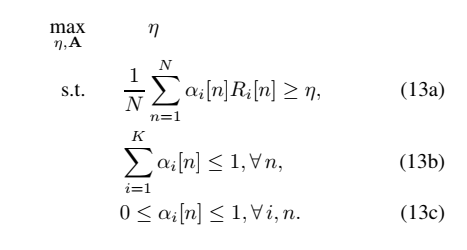# Is equal this code and this problem?

Hello, Is equal this code and this problem?
Thanks

problem:code:

``````cvx_begin
variable a(N,K) nonnegative
expression R(K,1);
expression r;
expression eta;
maximize eta
subject to
for i = 1 : K
r = 0;
for n = 1 : N
r = r + a(n,i) * log2(1+(gama0/(H^2+sum_square_abs(Q(n,:)-X(i,:)))));
end
R(i) = r / N;
end
eta <=  min(R) ;
sum(a,2) <= ones(N,1);
a <= ones(N,K);
cvx_end``````

I don’t see anything wrong with your code. Of course, you didn’t show the mathematical formulation of R_i[n], so I will defer to you on that. This is just a Linear Programming problem; so it is very straightforward to code, other than making sure indexing is done correctly.

Note that you can use `1` instead of `ones(N,K)` and `ones(N,1)` in the inequality constraints. But there is nothing wrong with using `ones`.